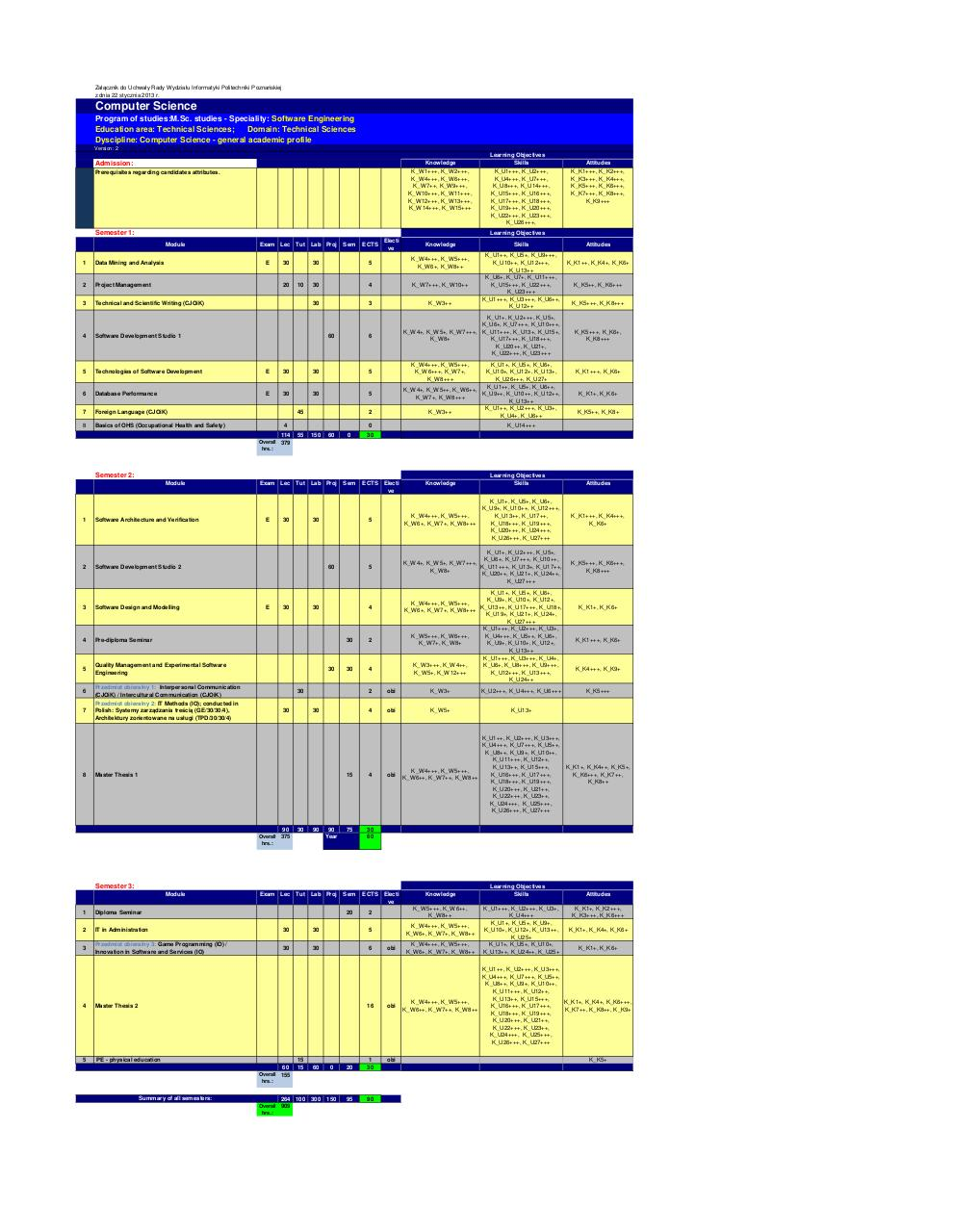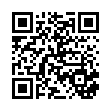# SE program ksztalcenia .pdf

### File information

Original filename: SE program ksztalcenia.pdf
Title: Computer Science-SE 2013.xlsx
Author: Filipiak

This PDF 1.3 document has been generated by PScript5.dll Version 5.2.2 / GPL Ghostscript 8.64, and has been sent on pdf-archive.com on 13/09/2015 at 16:30, from IP address 194.149.x.x. The current document download page has been viewed 432 times.
File size: 36 KB (2 pages).
Privacy: public file

SE program ksztalcenia.pdf (PDF, 36 KB)

### Document preview

Załącznik do Uchwały Rady Wydziału Informatyki Politechniki Poznańskiej
z dnia 22 stycznia 2013 r.

Computer Science
Program of studies:M.Sc. studies - Speciality: Software Engineering
Education area: Technical Sciences;
Domain: Technical Sciences
Dyscipline: Computer Science - general academic profile
Version: 2

Knowledge

K_W1+++, K_W2+++,
K_W4+++, K_W6+++,
K_W7++, K_W9+++,
K_W10+++, K_W11+++,
K_W12+++, K_W13+++,
K_W14+++, K_W15+++

Prerequisites regarding candidates attributes.

Semester 1:

Learning Objectives
Skills
K_U1+++, K_U2+++,
K_U4+++, K_U7+++,
K_U8+++, K_U14+++,
K_U15+++, K_U16+++,
K_U17+++, K_U18+++,
K_U19+++, K_U20+++,
K_U22+++, K_U23+++,
K_U26+++,

Attitudes
K_K1+++, K_K2+++,
K_K3+++, K_K4+++,
K_K5+++, K_K6+++,
K_K7+++, K_K8+++,
K_K9+++

Learning Objectives
Module

Exam Lec Tut Lab Proj
E

30

Sem

ECTS

K_W4+++, K_W5+++,
K_W6+, K_W8++

30

4

K_W7+++, K_W10++

30

3

K_W3++

Data Mining and Analysis

30

2

Project Management

3

Technical and Scientific Writing (CJOiK)

4

Software Development Studio 1

5

Technologies of Software Development

E

30

30

5

6

Database Performance

E

30

30

5

7

Foreign Language (CJOiK)

8

Basics of OHS (Occupational Health and Safety)

10

60

K_W4+++, K_W5+++,
K_W6+++, K_W7+,
K_W8+++

K_U1+, K_U5+, K_U6+,
K_U10+, K_U12+, K_U13+,
K_U26+++, K_U27+
K_U1++, K_U5+, K_U6++,
K_W4+, K_W5++, K_W6++,
K_U9++, K_U10++, K_U12++,
K_W7+, K_W8+++
K_U13++
K_U1++, K_U2+++, K_U3+,
K_W3++
K_U4+, K_U6++

2

4
150

60

0

Attitudes
K_K1++, K_K4+, K_K6+

K_K5++, K_K6+++
K_K5+++, K_K8+++

K_K5+++, K_K6+,
K_K8+++

K_K1+++, K_K6+

K_K1+, K_K6+
K_K5++, K_K8+

K_U14+++

0
55

Skills
K_U1++, K_U5+, K_U9+++,
K_U10++, K_U12+++,
K_U13++
K_U6+, K_U7+, K_U11+++,
K_U15+++, K_U22+++,
K_U23+++
K_U1+++, K_U3+++, K_U6++,
K_U12++

K_U1+, K_U2+++, K_U5+,
K_U6+, K_U7+++, K_U10+++,
K_W4+, K_W5+, K_W7+++, K_U11+++, K_U13+, K_U15+,
K_W8+
K_U17+++, K_U18+++,
K_U20++, K_U21+,
K_U22+++, K_U23+++

6

45

114

Knowledge

5

1

20

Electi
ve

30

Overall 379
hrs.:

Semester 2:
Module

1

Software Architecture and Verification

2

Software Development Studio 2

3

Software Design and Modelling

4

Pre-diploma Seminar

5

Quality Management and Experimental Software
Engineering

6

Przedmiot obieralny 1: Interpersonal Communication
(CJOiK) / Intercultural Communication (CJOiK)

7

Przedmiot obieralny 2: IT Methods (IO); conducted in
Polish: Systemy zarządzania treścią (GE/30/30/4),
Architektury zorientowane na usługi (TPD/30/30/4)

8

Exam Lec Tut Lab Proj

E

30

Sem

30

60

E

30

30

Master Thesis 1

90

30

90

Overall 375
hrs.:

90
Year

5

K_U1+, K_U2+++, K_U5+,
K_U6+, K_U7+++, K_U10++,
K_W4+, K_W5+, K_W7+++,
K_U11+++, K_U13+, K_U17++,
K_W8+
K_U20++, K_U21+, K_U24++,
K_U27+++

K_K5+++, K_K6+++,
K_K8+++

K_U1+, K_U5+, K_U6+,
K_U9+, K_U10+, K_U12+,
K_W4+++, K_W5+++,
K_U13++, K_U17+++, K_U18+,
K_W6+, K_W7+, K_W8+++
K_U19+, K_U21+, K_U24+,
K_U27+++
K_U1+++, K_U2+++, K_U3+,
K_W5+++, K_W6+++,
K_U4+++, K_U5++, K_U6+,
K_W7+, K_W8+
K_U9+, K_U10+, K_U12+,
K_U13++
K_U1+++, K_U3+++, K_U4+,
K_W3+++, K_W4++,
K_U6+, K_U8+++, K_U9+++,
K_W5+, K_W12+++
K_U12+++, K_U13+++,
K_U24++

30

4

2

obi

K_W3+

K_U2+++, K_U4+++, K_U6+++

4

obi

K_W5+

K_U13+

15

4

75

30
60

obi

Exam Lec Tut Lab Proj

Sem

1

Diploma Seminar

2

30

30

5

3

Przedmiot obieralny 3: Game Programming (IO) /
Innovation in Software and Services (IO)

30

30

6

4

Master Thesis 2

5

PE - physical education

20

ECTS Electi
ve

60

15
15

60

Summary of all semesters:

264 100 300
Overall 909
hrs.:

K_W4+++, K_W5+++,
K_W6+, K_W7+, K_W8++
obi

16

obi

obi

0

20

1
30

150

95

90

Overall 155
hrs.:

Knowledge
K_W5+++, K_W6++,
K_W8++

2

K_K1+, K_K6+

K_K1+++, K_K6+

K_K4+++, K_K9+

K_K5+++

K_U1++, K_U2+++, K_U3+++,
K_U4+++, K_U7+++, K_U5++,
K_U8++, K_U9+, K_U10++,
K_U11+++, K_U12++,
K_U13++, K_U15+++,
K_K1+, K_K4++, K_K5+,
K_W4+++, K_W5+++,
K_U16+++, K_U17+++,
K_K6+++, K_K7++,
K_W6++, K_W7++, K_W8++
K_U18+++, K_U19+++,
K_K8++
K_U20+++, K_U21++,
K_U22+++, K_U23++,
K_U24+++, K_U25+++,
K_U26+++, K_U27+++

Semester 3:
Module

Attitudes

K_K1+++, K_K4+++,
K_K6+

2

30

Learning Objectives
Skills

5

30

30

Knowledge

K_U1+, K_U5+, K_U6+,
K_U9+, K_U10++, K_U12+++,
K_W4+++, K_W5+++,
K_U13++, K_U17++,
K_W6+, K_W7+, K_W8+++
K_U18+++, K_U19+++,
K_U20+++, K_U24+++,
K_U26+++, K_U27+++

4

30

30

ECTS Electi
ve

K_W4+++, K_W5+++,
K_W6+, K_W7+, K_W8++

Learning Objectives
Skills
K_U1+++, K_U2+++, K_U3+,
K_U4+++
K_U1+, K_U5+, K_U9+,
K_U10+, K_U12+, K_U13++,
K_U25+
K_U1+, K_U5+, K_U10+,
K_U13++, K_U24++, K_U25+

Attitudes
K_K1+, K_K2+++,
K_K3+++, K_K6+++
K_K1+, K_K4+, K_K6+
K_K1+, K_K6+

K_U1++, K_U2+++, K_U3+++,
K_U4+++, K_U7+++, K_U5++,
K_U8++, K_U9+, K_U10++,
K_U11+++, K_U12++,
K_U13++, K_U15+++,
K_W4+++, K_W5+++,
K_K1+, K_K4+, K_K6+++,
K_U16+++, K_U17+++,
K_W6++, K_W7++, K_W8++
K_K7++, K_K8++, K_K9+
K_U18+++, K_U19+++,
K_U20+++, K_U21++,
K_U22+++, K_U23++,
K_U24+++, K_U25+++,
K_U26+++, K_U27+++
K_K5+

Summary of the Program of Studies
Overall hours - summary of all semesters: 909
Consultations, exams: 217
All contact hours with tutors: 1 126
Required number of contact hours with tutors for full-time
1 125
studies 0.5*(90p.ECTS*25)
ECTS:
90
ECTS for elective modules:
33
Required number of hours in elective modules 30% from
27
90
Total number of hours within tutorials, laboratory classes,
645
project classes, and seminars:#### HTML Code

Copy the following HTML code to share your document on a Website or Blog

#### QR Code### Related keywords